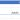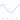# Course 1 Final Assessment >> Excel Skills for Business: Essentials

## Course 1 Final Assessment >> Excel Skills for Business: Essentials

1. Download the workbook and save it on your computer. Open the workbook and then come back here to start answering the questions.
C1 Assessment Workbook.xlsxTIP: Make sure that you save your progress on the workbook in Excel frequently as you progress through the exam.
Here is the first question:
The workbook contains data downloaded from a government database. It is not very easy to read, so your first job is to address some of the formatting.
The heading in cell A1 cannot be seen properly. Change the alignment so that you can see what it says.
In which year was this data collected?
Enter your answer in four digits ####

`2015`

2. Somehow the date in cell B2 has lost its formatting. Change the format to a date. What date is now shown in B2?
Enter your answer in Year-Month-Day format (e.g., 2016-12-31).

`2016-03-20`

3. Apply the style Accent1 to the range A2:Z2. Apply the style Heading 3 to the range A4:Z4. Which of the following looks most like your data?

• This:
• This:This One ✅ ( Check The Fonts Color on the picture. Full black one is not correct )

• This:
• This:

4. There are also some corrections you need to make to the data. One of the data entries is missing. You have been asked to Insert a row after Case ID 49 (row 15) and enter the following data:
Case ID 51Economic Position Full-time workingOccupation Type Managerial or ProfessionalHome OwnedGender MaleRegion WalesAdults 2Children 2Jan Income 5924.00Feb Income 5924.00Mar Income 5924.00Apr Income 5924.00May Income 5924.00Jun Income 5924.00Jan Expenditure 2803.57Feb Expenditure 2242.86Mar Expenditure 2512.00Apr Expenditure 2361.28May Expenditure 2219.60Jun Expenditure 2596.93What is the total April Expenditure now? (cell R2)
In your answer, you should use the number format #####.## with a period as a decimal point and two decimals.

`4534401.10`

5. An incorrect value has been entered for Case ID 5299. Use the Find tool to find this Case ID and change the January Income to \$200. What is the total January Income now? (cell I2)
In your answer, you should use the number format #####.##

`6074344.46`

6. There are several calculations missing which need to be added. An additional column showing the total number of people per household is required. Perform all the following steps and then answer the question.
Insert a column after column HIn I4 type the heading HouseholdIn I5 enter a calculation to add the number of adults in G5 to the number of children in H5Copy the formula down to fill the columnIn cell I2, enter a calculation to get the total number of people in all the households represented, or copy the formula across from cell H2QUESTION: What is the total Household value in cell I2?
Enter your answer in numerical digits: ######

`6378`

7.In cell V5 enter a calculation to get an average of income from January to June (J5 to O5). Copy the formula down the column. What is the Average Income for Case 15 (cell V8)?
In your answer, you should use the number format #####.##

`4019.36`

8. In cell W5 enter a calculation to add up the total income from January to June. Copy the formula down the column. Widen the column so that you can see the results. What is the total income for all the cases (cell W2)?
In your answer, you should use the number format #####.##

`19943.28`

9. In cell X5 enter a calculation to add up the total expenditure from January to June (P5 to U5). Copy the formula down the column. What is the total expenditure for Case 9?
In your answer, you should use the number format #####.##

`10709.83`

10. In cell Y5 enter a calculation to subtract Total Expenditure from Total Income. Copy the formula down the column. What is the Net for Case 9?
In your answer, you should use the number format #####.##

`17423.96`

11. Cost of living has been estimated at going up by 3.93% over the next 6 months. We would like to forecast what the expenditure will be over that period. In cell Z2 enter the value 3.93%. In Z5 enter a formula to calculate the forecast expenditure. To do this you will need to calculate the increase in expenditure (current total expenditure multiplied by the percentage increase) and add it to the current total expenditure. Copy the formula down the column. (Make sure that all the calculations are using the value in Z2!).
QUESTION: What is the Forecast Expenditure for Case 15?
In your answer, you should use the number format #####.##

`13256.09`

12. Now select the Stats Worksheet. Enter simple formulas in B3 and B4 to pull through the calculated Total Expenditure and Total Net from the Data worksheet (cells X2 and Y2). If you have done it correctly the pie chart should now show how income is proportioned between expenditure and net.
QUESTION: According to the pie chart, what percentage of Income is made up by Expenditure?
Do not enter the % symbol in the answer box below, just the number.

`25`

13. Still on the Stats sheet, enter a formula in B5 to add up the total income for Quarter 1 using the calculated totals for January, February and March in the Data worksheet. If you have done it correctly the cell should change colour.
QUESTION: What colour is the cell B5?

• Black
• Yellow
• White
• Grey
• Purple

14. The organisation has decided to have one Region for the Midlands instead of two, so both East Midlands and West Midlands need to be replaced with just Midlands. We then need to answer some questions for the organisation.
In the Data worksheet, use Find and Replace to replace all instances of East Midlands with Midlands. Repeat the operation, this time replacing West Midlands with Midlands. Now filter the data so that only cases from the Midlands are visible.
QUESTION: What is the total number of Adults recorded for the Midlands?

`476`

15. Clear the previous filter. Add filters so that we only see cases for Wales with 6 or more people in the household.
QUESTION: How many households in Wales have 6 or more people?

`1`

16. Clear all filters. Sort the data by Total Income in descending order (largest to smallest).
QUESTION: Which Case ID has the highest Total Income?

`40001.26`

17. Change the sort to order the data so that you can easily identify the lowest Average Income for Cases with an Intermediate occupation.
QUESTION: What is the lowest Average Income for people with an Intermediate occupation?
In your answer, you should use the number format #####.##

`18.35`

18. You are concerned there may be duplicates in the data set. Add conditional formatting to the Case ID column to show all duplicates in red. Sort the data by Case ID but instead of by values, sort by colour.
QUESTION: How many cases have been duplicated (entered twice)?

• 2
• 3
• 5
• 6

19. Delete one of each of the duplicate rows.
QUESTION: What is the new total in G2?

`3513`

20. To help represent the data graphically you have been asked to create a few charts. You will need to go back to the Stats worksheet.
Select the data from A8 to B12. Insert a Pie Chart to compare the Average Incomes for different Economic Positions. Add a quick layout that shows a percentage for each segment.
QUESTION: What is the percentage for Economically Inactive?
Do not enter the % symbol in the answer box below, just the number.

`63`

21. Create a line chart showing the Total Income for each Month. Ensure you select month names and Total Income values.
QUESTION: Which of these charts looks most like your line chart?

• This:
• This:
• This:This One ✅

22.Insert a Stacked Column Chart to show the Jan, Feb and Mar income for each Region. Which region has the second lowest income for Jan-Mar (second smallest stack)?

`Wales`